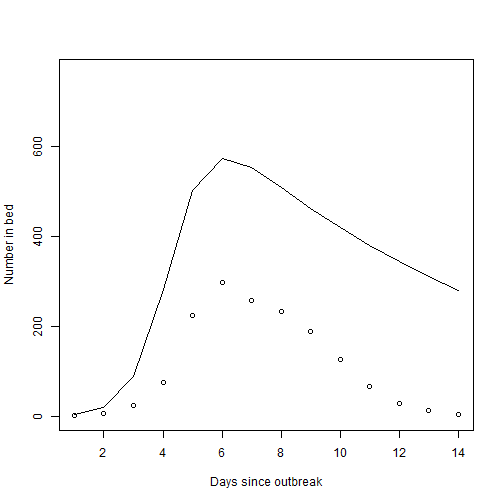# Description of the SIR model for a disease outbreak

## Description of the data

We will use the Influenza in a boarding school in England, 1978 in the packages outbreaks for illustration. The data are described in the packages as:

These data comprise of a time series of influenza cases in a boarding school in England. The original data were available only in a figure with some additional data in the main text; hence, the exact numbers vary depending on the source. These data are from Chapter 9 of De Vries et al. (1996). The index case was infected by 1978-01-10, and had febrile illness from 1978-01-15 to 1978-01-18. 512 boys out of 763 became ill.

Example model fits are given below, and interactive model fitting can be performed at

https://shiny.stat.ncsu.edu/bjreich/SIR/

library(outbreaks)
influenza_england_1978_school

##          date in_bed convalescent
## 1  1978-01-22      3            0
## 2  1978-01-23      8            0
## 3  1978-01-24     26            0
## 4  1978-01-25     76            0
## 5  1978-01-26    225            9
## 6  1978-01-27    298           17
## 7  1978-01-28    258          105
## 8  1978-01-29    233          162
## 9  1978-01-30    189          176
## 10 1978-01-31    128          166
## 11 1978-02-01     68          150
## 12 1978-02-02     29           85
## 13 1978-02-03     14           47
## 14 1978-02-04      4           20

Y  <- influenza_england_1978_school[,2]
nt <- length(Y)     # Number of time sets
N  <- 763           # Population size
I0 <- 1             # Initial number of infected

plot(Y,ylim=c(0,N),xlab="Days since outbreak",ylab="Number in bed")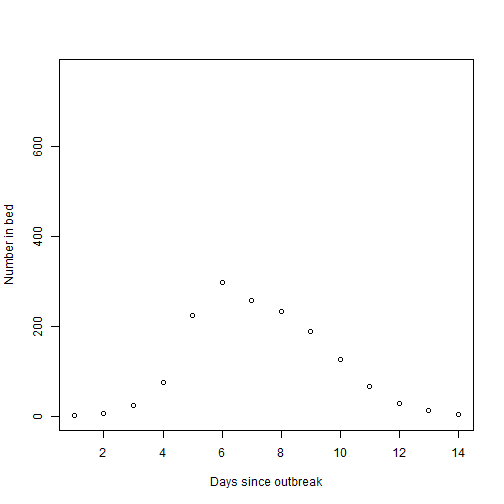## Description of the SIR model

The Susceptible-Infected-Recovered (SIR) model is one of the most basic models of disease spread. At time $$t$$, let $$S_t$$, $$I_t$$ and $$R_t$$ be the number of people in each condition, with $$S_t+I_t+R_t=N$$ for all $$t$$. The states evolve according to the differential equations $\frac{dS_t}{dt} = -\beta S_tI_t/N \hspace{36pt} \frac{dI_t}{dt} = \beta S_tI_t/N - \gamma I_t.$ The parameter $$\beta$$ controls the rate of new infections and the parameter $$\gamma$$ controls the recovery rate. We will use a discrete approximation to these curves with hourly time steps, so $$dt = 1/24$$. Here are the curves for a few values of the parameters $$\beta$$ and $$\gamma$$.

SIR <- function(I0,N,beta,gamma,nt,m){
# Initial states
i  <- I0
s  <- N-I0
dt <- 1/m
I  <- rep(0,nt)

for(t in 1:nt){
for(j in 1:m){
s <- s - dt*beta*i*s/N
i <- i + dt*beta*i*s/N - dt*gamma*i
}
I[t] <- i
}
return(I)}

# Reasonable fit
beta  <- 1.7
gamma <- 0.5
I     <- SIR(I0=I0,N=N,beta=beta,gamma=gamma,nt=nt,m=24)
plot(Y,ylim=c(0,N),xlab="Days since outbreak",ylab="Number in bed")
lines(I)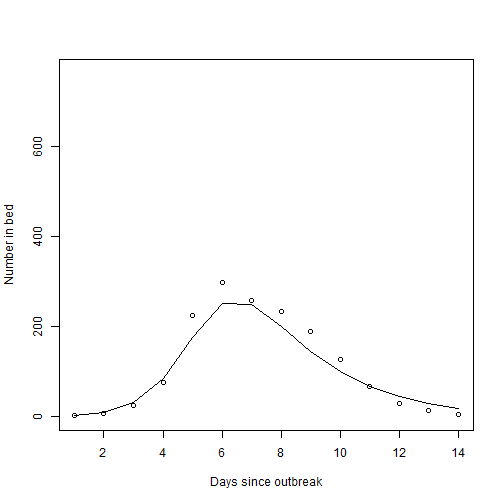## Larger infection rate

Increasing $$\beta$$ gives a steeper curve because the disease spreads faster.

beta  <- 2.0
gamma <- 0.5
I     <- SIR(I0=I0,N=N,beta=beta,gamma=gamma,nt=nt,m=24)
plot(Y,ylim=c(0,N),xlab="Days since outbreak",ylab="Number in bed")
lines(I)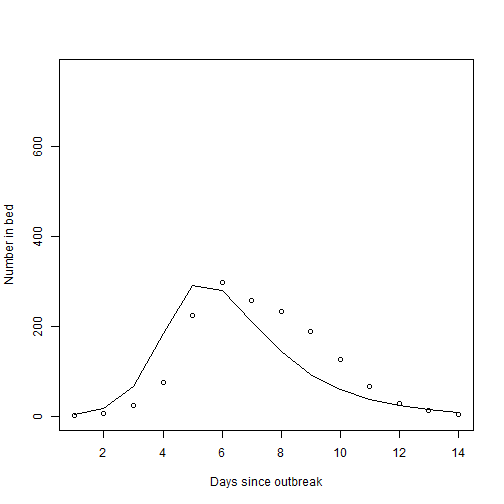## Smaller infection rate

Decreasing $$\beta$$ flattens the curve.

beta  <- 1.0
gamma <- 0.5
I     <- SIR(I0=I0,N=N,beta=beta,gamma=gamma,nt=nt,m=24)
plot(Y,ylim=c(0,N),xlab="Days since outbreak",ylab="Number in bed")
lines(I)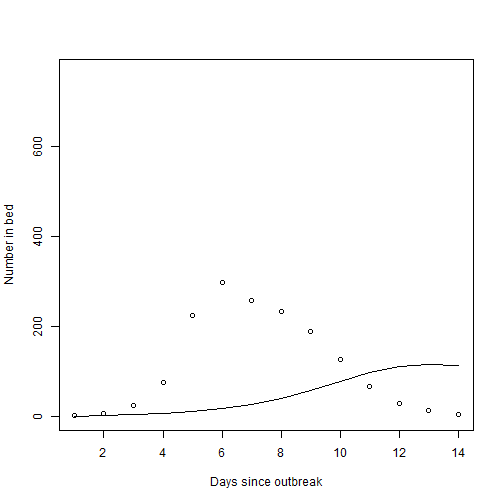## Larger recovery rate

Increasing $$\gamma$$ gives fewer overall infections because people are infectious for a shorter amount of time.

beta  <- 1.7
gamma <- 1.0
I     <- SIR(I0=I0,N=N,beta=beta,gamma=gamma,nt=nt,m=24)
plot(Y,ylim=c(0,N),xlab="Days since outbreak",ylab="Number in bed")
lines(I)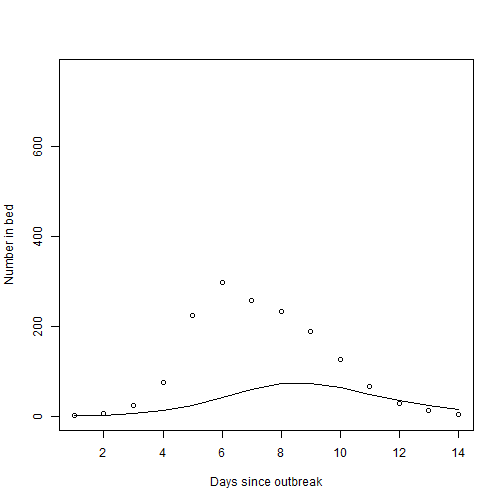## Smaller recovery rate

Decreasing $$\gamma$$ gives more overall infections because people are infectious for a longer amount of time.

beta  <- 1.7
gamma <- 0.1
I     <- SIR(I0=I0,N=N,beta=beta,gamma=gamma,nt=nt,m=24)
plot(Y,ylim=c(0,N),xlab="Days since outbreak",ylab="Number in bed")
lines(I)